# C/C++知识总结

1.数组
2.指针
3.存储
4.结构体
5.const修饰符
6.引用
7.作用域
8.重载
9.封装
10.初始化列表
11.static关键词
12.友元
13.继承
14.多态
15.封装

1.数组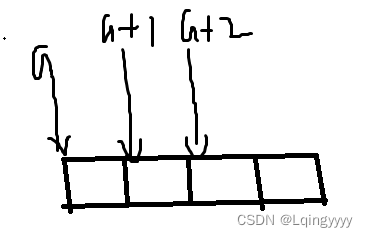int a;
a = 1;
cout << *(int *)((char *)&a) << " " << *(int *)((char *)&a + 1) << " " << *(int *)((char *)&a + 2) << " " << *(int *)((char *)&a + 3) << endl;
cout << a << " " << &a << " " << &a << " " << (a + 2) << endl;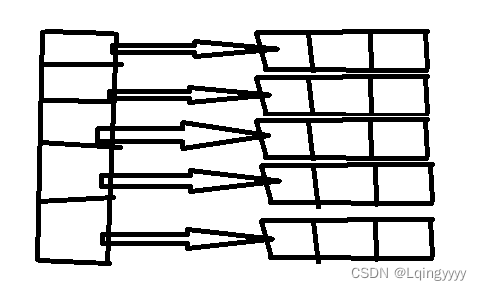2.指针

1. type *a 是一级指针 表示 a是一个指针 指向type类型的地址想要获取a的值就需要解引用 *a即可 那么对于 type **a 是二级指针 表示 a是一个指针 指向 指向type类型的指针地址的地址 简化以下就是 a是一个指针 指向 一个type类型指针的地址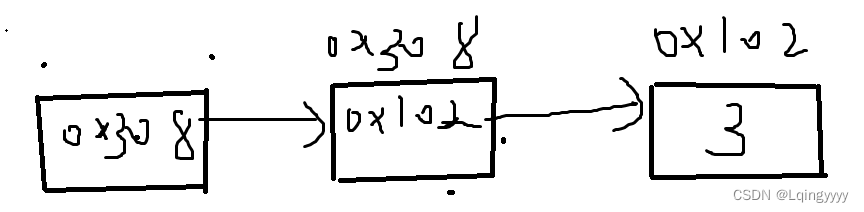2. 对于有数组的指针来说 一般是会先和数组结合 例如 int *a a就会和先结合 表示 a是一个有5个元素的数组 每个元素存的是 int类型的指针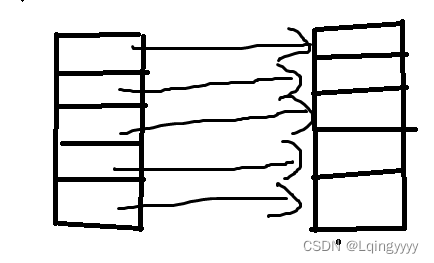那么如果是 int (a) 表示 a是一个指针 指向 int类型数组的首地址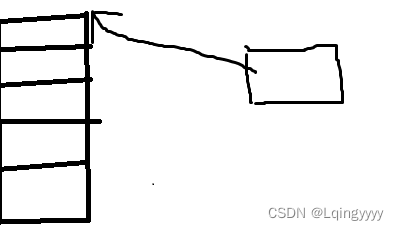函数指针 type (
) () 这样形式的就是一个函数指针 最右边的表示所拥有的变量 例如 int (*a)(int x,int y) 表示a是一个指针指向函数地址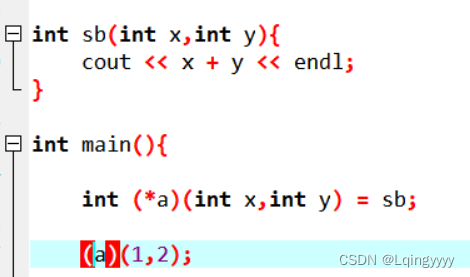对于上述图片来说 指向了 sb函数的地址 函数的名字代表函数的地址 在下边运用的时候 你可以解引用也可以不解引用 那么对于 int(**a)(int x,int y) 去掉了 a来说剩下的就是 int()(int x,int y) 代表的就是 a是指针 指向了一个函数指针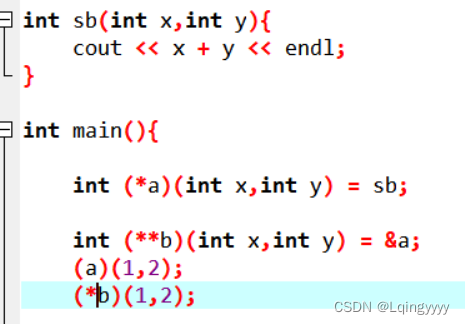对于b来说必须解引用 因为解引用了之后才能表示他是一个函数指针

3.存储
局部变量存在栈区 static和全局变量是放在静态区的 无论是全局static还是局部都是在静态区 常量存在常量区 堆区需要我们自己分配 例如 malloc 和 free 不过记得释放

4.结构体
1.第一个成员在结构体变量偏移量为0的地址处。

2.其他成员变量要对齐到某个数字（对齐数）的整数倍的地址处。

3.结构体总大小为最大对齐数的整数倍（每个成员变量都有一个对齐数）。

4.如果嵌套了结构体，嵌套的结构体对齐到自己最大对齐数的整数倍处，结构体的整体大小就是所有所有最大的对齐数的整数倍（包含嵌套结构体的对齐数）。

5.const修饰符解析
const 会对于 就近的数修饰为常量

const int *a 修饰了 *a为常量但是 a不是常量 所以a地址可修改
const int * const a 表示 *a和 a都是常量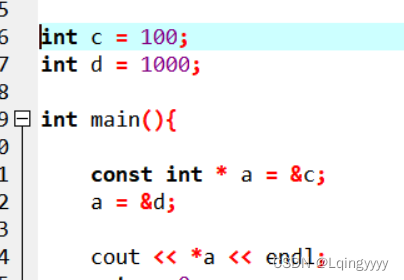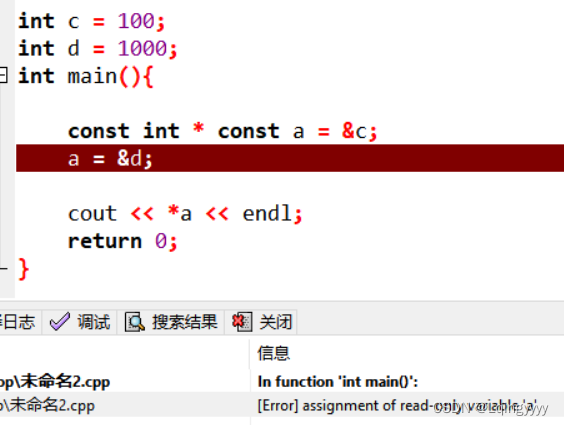6.引用

7.作用域
1、首先对于创建的变量来说，有两个属性特别重要：
（1）作用域 （2）以及生命周期
2、一般，作用域一般分为全局作用域、局部作用域、类作用域、语句作用域、文件作用域以及命名作用域；

3、

8.重载

1. 函数重载
函数重载对于同一个作用域下相同变量名的函数只需要满足以下一点即可 1.变量个数不同2.变量顺序不同3.函数参数类型不同 就可以进行重载了
2.运算符重载 要使用 operator 我分别举几个例子吧
加法运算符重载:
struct node{
int x,y;
node operator+(node &a){
node d = {this->x + a.x,this->y + a.y};
return d;
}
};


//写在结构体外
ostream & operator<<(ostream &cout,node &a){
printf("%d %d\n",a.x,a.y);
return cout;
}
//写在结构体内
firend ostream & operator<<(ostream &cout,node &a){
printf("%d %d\n",a.x,a.y);
return cout;
}
//为什么我要写返回的类型为 ostream & 因为为了保证程序的健壮性 cout << a << endl; 前面 cout << a如果返回的不是 &cout类型那么后续就会报错


node & operator++(){
this->x++;
return *this;
}


node & operator++(int ){
int x = this->x;
this->x += 1;
node s = {x,this->y};
return s;
}
//后面的int表示占位 可以区分前置++


9.封装

• class代表封装 和结构体有点相似但是结构体默认全是public
注意1.:成员函数和成员变量是分开存的所以class里面的内存大小只有成员变量(非静态) 如果没有变量的话 默认占一个内存空间
注意2.成员函数如果是个虚函数的话 内部会存在一个4个字节的vfptr(virtual function pointer)指针指向vftable虚函数表 表内部记录虚函数的地址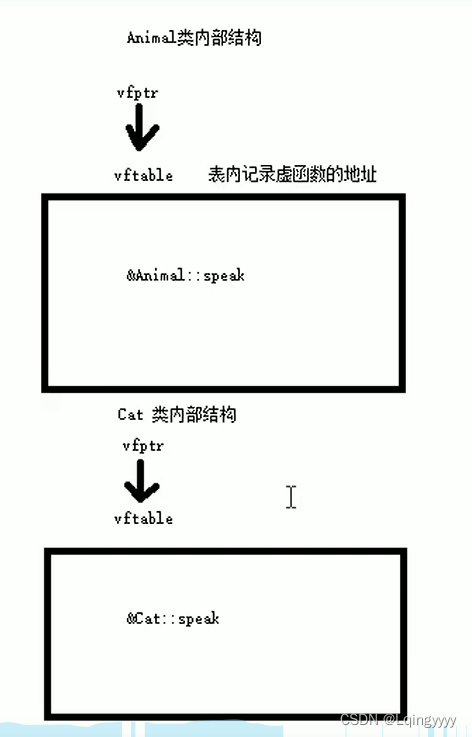class可以更改权限:

class{
public: int a;
private: int b;
};
//这样外部访问不到b这个值 只能内部访问


• 构造函数与拷贝构造函数与析构函数
构造函数的调用规则 :
默认构造函数 (无参，函数体为空)
默认拷贝构造函数(对属性值进行拷贝)
如果构造了有参构造函数 默认无参构造函数消失 但是会提供默认拷贝函数
如果构造了默认拷贝函数 系统不会提供其他构造函数
//构造函数和拷贝构造都是无返回值
class ss{
public:
int a,b;

ss(){ //无参构造

}
ss(int a){ //有参构造
this->a = a;
}
ss(const ss &p){ //拷贝构造
this->a = p.a;
this->b = p.b;
}
//注意拷贝对象不要初始化匿名对象
//ss s3;
// ss(s3) == ss s3; 所以会报错

//隐式转换法 ss s3 = 10 与 ss s3 = ss(10)
};


1.使用已经初始化过的对象来创建另一个对象
2.值传递的方式给函数传参
3.以值方式返回给局部变量

10.初始化列表

class node{
public:
int a,b;
int *c;

node():a(10),b(20){
}
node(int a,int b):a(a),b(b){

}
};


11.static

12友元

• 全局函数做友元 函数可以访问class里面的私密成员
• 类做友元 另一个类产生的对象可以访问当前类的私密成员
• 成员函数做友元 该函数可以访问当前类的私密成员

13.继承
1.继承的第一大特点就是提高代码复用性
2.继承的权限: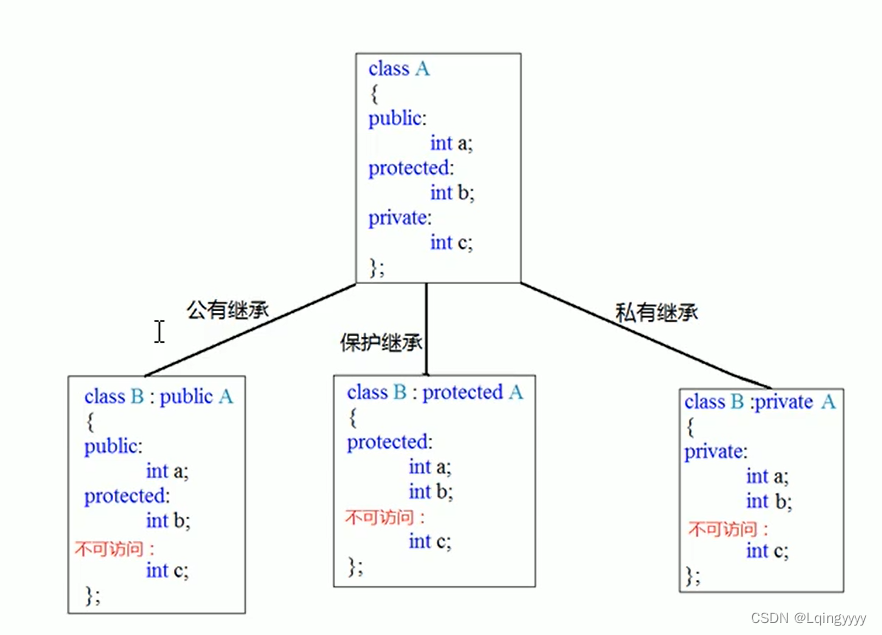3.继承后子类有哪些成员

4.继承中构造与析构的关系

5.继承中同名的访问方式

6.菱形继承的处理方式 (继承多个爹 但是爹的爹是一样的产生了重复的属性)

14.多态
1.静态多态与动态多态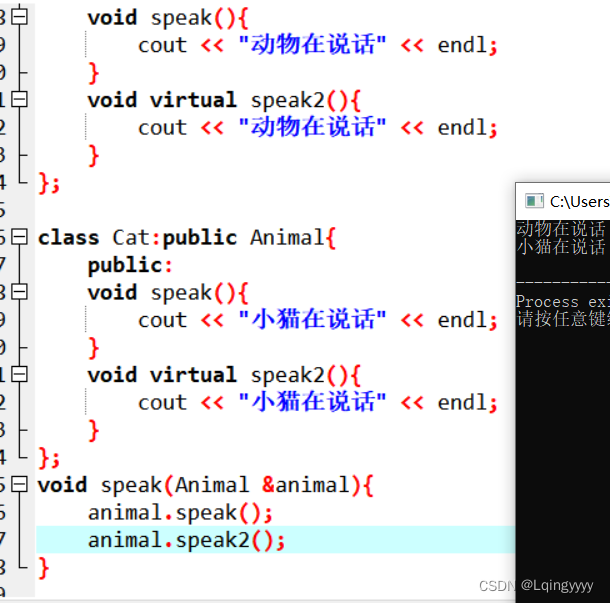15.模板

• 函数模板:
template<typename T,typename TT> typename可以换成class
void 函数名（T a,TT b)
//使用事项 1.系统自动推导出T等typename才能使用
//2.函数名<type,type>()自己写出T等typename代表的类型


#define Template template
#define Typename typename
Template <Typename T>
bool myswap(T& a, T& b) {
if (a == b) return true;
else return false;
}
//如果T传的是一个结构体他就不知道如何去比较
//那么我们必须重载模板
Template <>
bool myswap(node& a, node& b) {
if (a.x == b.x && a.y == b.y) return true;
else return false;
}

• 类模板
Template <Typename T>
class 类名<Typename a,Typename b{
void sb(T &a);
}

Template<Typename T>
void 类名<T>::sb(T &a){
} //类外实现


Template <Typename T = int>
class 类名<Typename a,Typename b{

}


1.指定传入类型

Template <Typename T>
class node{
public:
T x, y;
};

void show(node<int> &a) {
cout << a.x << " " << a.y;
}

int main() {
node<int> a = { 1,2 };
show(a);



2.参数模板化

Template <Typename T>
class node{
public:
T x, y;
};

Template <Typename T>
void show2(node<T>& a) {
cout << a.x << " " << a.y;
}
node<int> a = { 1,2 };
show2(a);


3.整个类模板化

Template <Typename T>
class node{
public:
T x, y;
};

Template <Typename T>
void show2(T& a) {
cout << a.x << " " << a.y;
}
node<int> a = { 1,2 };
show2(a);


1.儿子继承父类要把父模板的类型指定出来

class node{
public:
T x, y;
};

class node2 :public node<int> {

};


2.想灵活指定父亲模板的类型 需要把儿子也变成模板

Template <Typename T>
class node{
public:
T x, y;
};

Template <Typename T,Typename T2>
class node3 : public node <T> {

};


1.首先类模板里面的实现函数如果包含Typename的话他是不会直接创建出来的所以我们如果分.h 和 .cpp去写的类模板 我们需要直接包含.cpp 因为.h不创建出来所以没有函数声明
2.将.h和.cpp写在一起 .hpp文件表面这是一个类模板文件

1.全局函数内部实现

Template <class T>
class node{
friend void awr(node &a) {
cout << a.x  << endl;
}
public:
node(int x, int y) {
this->x = x, this->y = y;
}
private:
T x, y;
};


2.全局函数外部实现

//首先声明这个类
Template<class T>
class node;
//声明这个模板的实现形式
Template<class T>
void awr(node<T> a) {
cout << a.x <<  " " << a.y << endl;
}

Template <class T>
class node{
//友元awr是个模板
friend void awr<T>(node<T> a);
public:
node(int x, int y) {
this->x = x, this->y = y;
}
private:
T x, y;
};


11-05
09-05
08-02
01-13706
02-08911
09-26272
09-1911万+
09-27245
08-104万+
02-04133
09-222万+
10-18690
09-241205
04-224874
08-30570
07-122495
11-03

### “相关推荐”对你有帮助么？

•非常没帮助
•没帮助
•一般
•有帮助
•非常有帮助被折叠的  条评论 为什么被折叠?到【灌水乐园】发言Lqingyyyy

¥2 ¥4 ¥6 ¥10 ¥20余额支付 (余额：-- )扫码支付获取中扫码支付点击重新获取扫码支付1.余额是钱包充值的虚拟货币，按照1:1的比例进行支付金额的抵扣。
2.余额无法直接购买下载，可以购买VIP、C币套餐、付费专栏及课程。余额充值